# 逆向脱壳分析基础学习笔记五 标志寄存器

## EFLAGS寄存器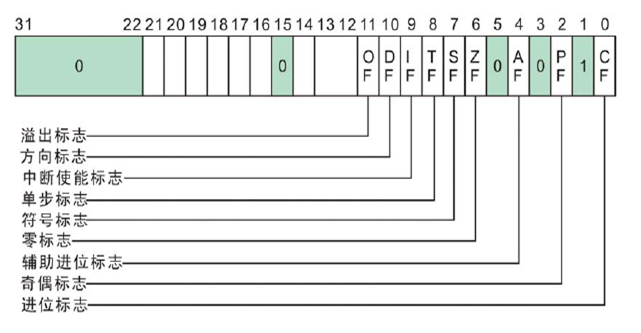## 进位标志CF(Carry Flag)

``````MOV AL,0xFF

``````

0x80+0x40

0x80:0 1000 0000

0x40:0 0100 0000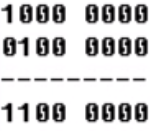### 0x80-0x40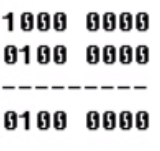### 0x80-0x81

0x80:1000 0000

0x81:1000 0001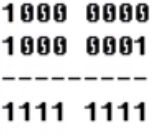## 奇偶标志PF(Parity Flag)``````MOV AX,803
``````

0x803: 0000 1000 0000 0011

0x804: 0000 1000 0000 0100 总共2个1 ,PF应为1，但实际运行结果PF为0

04： 0000 0100 总共1个1，所以PF为0

## 辅助进位标志AF(Auxiliary Carry Flag)

• 在字操作时，发生低字节向高字节进位或借位时
• 在字节操作时，发生低4位向高4位进位或借位时

AF与数据宽度相关

32位时 FFFF F FFF

16位时 FF F F

8位时 F F

32位例：

``````MOV EAX,55EEFFFF

``````

16位例：

``````MOV AX,5EFE

``````

8位例：

``````MOV AL,4E

``````

## 零标志ZF(Zero Flag)

``````XOR EAX,EAX
``````

``````MOV EAX,0
``````

## 符号标志SF(Sign Flag)

``````MOV AL,7F
``````

## 溢出标志OF(Overflow Flag)

• 正 + 正 = 正 如果结果是负数，则说明有溢出
• 负 + 负 = 负 如果结果是正数，则说明有溢出
• 正 + 负 永远都不会有溢出

### 无符号、有符号都不溢出例

``````MOV AL,8
``````

AL的数据宽度为8，即

8+8=16在0~255内 不溢出

8+8=16 在0~127内 两正数相加结果仍为正数，不溢出

### 无符号溢出、有符号不溢出例

``````MOV AL,0FF
``````

FF+2=255+2=257 在0~255外，溢出

FF+2=-1+2=1

### 无符号不溢出、有符号溢出例

``````MOV AL,7F
``````

7F+2=127+2=129 在0～255内 不溢出

7F+2=0x81在80～FF (负数范围)内，两正数相加结果为负数，溢出

### 无符号、有符号都溢出

``````MOV AL,0FE
``````

FE+2=254+2=256=0x100 在0～255外 溢出

FE+2=0x100在0~FF外，溢出

### CPU如何计算OF位

• 符号位有进位
• 最高有效数值位向符号位产生的进位

0x80:1 000 0000

0xC0:**1 **100 0000

0x80:1 0 00 0000

0xC0:1 1 00 0000

``````MOV AL,80
``````

0x80:1 0 00 0000

0xC0:1 1 00 0000

OF = 符号位有进位 xor 最高有效数值位向符号位产生的进位

OF = 1 xor 0 = 1 所以此时OF=1

## 方向标志DF(Direction Flag)

DF：方向标志位

DF=1时串操作为减地址方式 DF=0为增地址方式

## 相关汇编指令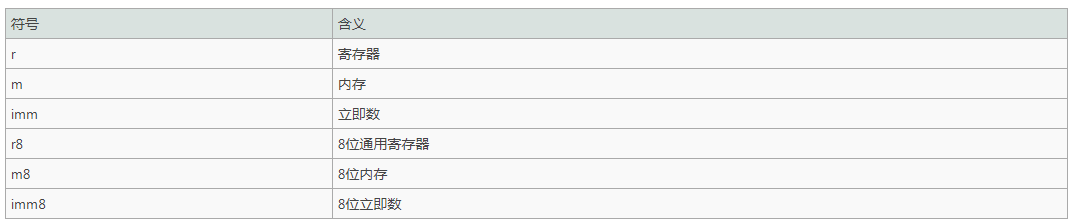``````MOV AL,1
MOV CL,2

``````

### SBB指令：带借位减法

``````MOV AL,4
MOV CL,2

SBB AL,CL
``````

### XCHG指令：交换数据

``````XCHG AL,CL
XCHG DWORD PTR DS:[12FFC4],EAX
XCHG BYTE PTR DS:[12FFC4],AL
``````

``````MOV AL,1
MOV CL,2
XCHG AL,CL
``````

### MOVS指令：移动数据 内存-内存

BYTE/WORD/DWORD

MOVS指令常用于复制字符串

``````MOVS BYTE PTR ES:[EDI],BYTE PTR DS:[ESI]        简写为：MOVSB
MOVS WORD PTR ES:[EDI],WORD PTR DS:[ESI]        简写为：MOVSW
MOVS DWORD PTR ES:[EDI],DWORD PTR DS:[ESI]        简写为：MOVSD
``````

``````MOV EDI,12FFD8
MOV ESI,12FFD0
MOVS DWORD PTR ES:[EDI],DWORD PTR DS:[ESI]
``````

### STOS指令

``````STOS BYTE PTR ES:[EDI]                将AL存储到[EDI]
STOS WORD PTR ES:[EDI]                将AX存储到[EDI]
STOS DWORD PTR ES:[EDI]                将EAX存储到[EDI]
``````

### REP指令

``````MOV ECX,10
REP MOVS DWORD PTR ES:[EDI],DWORD PTR DS:[ESI]        也可以写成REP MOVSD
``````